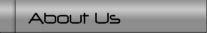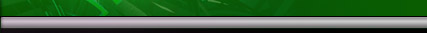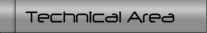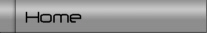# .: series & parallel circuits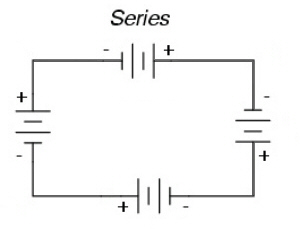With batteries in series the voltage of the batteries are added, but the Amp hour rating (Ah) is not combined. The voltages can be different, but the Ah should be the same, otherwise the lowest Ah battery will reduce the Ah rating of the entire pack.When batteries are connected in parallel the voltage of the pack does not change, but the Ah rating of each battery are added. Each battery should have the same voltage. The Ah can be different, but doing so will stress the lowest Ah battery and reduce its life.

# .: power (watts)

Watts = volts * amps

# .: current capacity for copper

The current capacity of a conductor is limited by the amount of heat it can withstand. The general rule of thumb for a copper conductor with continuous DC current, is 1000 amps per square inch. AC current does not generate as much heat so its generally accepted guidelines are 1300 amps per square inch.

# .: diodes

Diodes are one-way valves. They allow current to flow in one direction, but not the other.

# .: fuses

Fuses limit the current flowing in a circuit. For low voltage devices (below 50 volts), the voltage rating of a fuse is not an issue. However, when voltages higher than a fuses rating blows, a spark may jump between the contacts which allows current to flow in the circuit.

# .: fusing a dc-dc converter

A typical electric vehicle's 400 watt dc-dc converter will supply 30 amps at 12.5 volts (30 amps * 12.5 volts = 375 watts). The output should be fused at 30 amps, but if its input is 150 volts, the input fuse only needs to be 3 amps, NOT 30amps!  This is because the converter has 400 watts input:

• 400 watts / 150 volts = 2.667 amps

# .: resistors

Resistors are called a passive device because it there is no method to control its behavior. It reduces the current and voltage (power) to the circuit downstream. In exchange for this reduction in power, it give off heat.

# .: transformers (dc-dc converter)

There are a multitude of transformers. Transformers do not increase the power, they change it by either increasing the available voltage and decreasing the available amperage (like an ignition coil), or decreasing the available voltage and increasing the available amperage (like electrical grid transformers).

For electric vehicles, most transformers step-down the voltage to supply the typical 12 volt devices. A dc-dc converter is rated in watts (volts * amps). Due to inefficiencies, more watts go into a converter than come out.